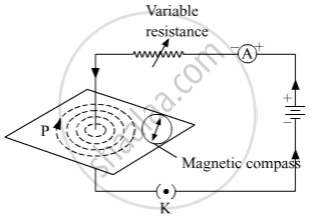# How Will the Strength of the Magnetic Field Change When the Point Where Magnetic Field is to Be Determined is Moved Away from the Straight Wire Carrying Constant Current? Justify Your Answer. - Science

#### Question

How will the strength of the magnetic field change when the point where the magnetic field is to be determined is moved away from the straight wire carrying constant current? Justify your answer.

#### Solution

Using a compass needle we can determine the magnetic field. When we move away from the compass needle from the straight wire, the deflection of the needle decreases which implies the strength of the magnetic field decreases, as the strength of magnetic field produced by a straight wire at any point is inversely proportional to the distance of the point from the wire.Is there an error in this question or solution?